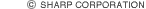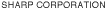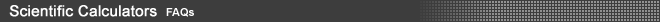Here are links to frequently asked questions. For your convenience, these questions have been divided into two categories. General Questions deals with questions concerning scientific calculators in general while Questions Concerning Operation answers questions related specifically to operating our calculators.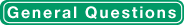What is a WriteView calculator?A WriteView calculator is a scientific calculator that can display fractions and symbols (such as “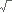”) that are used in mathematical formulas, as in the display example shown here.What is a scientific calculator? Calculators that have functions such as trigonometric functions are generally called scientific calculators.What is a programmable calculator? A programmable calculator is a scientific calculator with algebraic memory functions.What is a graphing calculator? A graphing calculator is a scientific calculator with graphing functions.What is the D.A.L. system? D.A.L. is the abbreviation for the Direct Algebraic Logic system. It is a way of inputting that follows the exact order of the equation. Sharp was the first company to incorporate this system into non-programmable scientific calculators in 1992.How is the D.A.L. system different from V.P.A.M.? V.P.A.M. can be found on Casio's calculators and is essentially the same as Sharp's D.A.L. Nowadays it has become standard to enter an equation as it appears on the page.What should I pay attention to when buying a new scientific calculator? Scientific calculators have many functions. Therefore, you should know what functions are needed today or in the future and whether the functions in your field are included. Other factors, such as price, durability and usability, should also be considered.Are the functions and operating methods different between educational and general scientific calculators? Basically they are the same. Educational calculators have functions that are helpful for education, such as the D.A.L. system and the mathematical display.Why are scientific calculators used in education? Many students say they don't like mathematics or science and find calculations difficult. Scientific calculators reduce the burden of computation and allow teachers to spend more time explaining theories and showing fascinating aspects of mathematics, thereby helping students become interested in mathematics. This is why the use of calculators in classes and in tests has been spreading world-wide.Tell me about cheating prevention and the model certification system. In order to prevent cheating, some schools ban programmable and graphing calculators that can store formulas or functions that interfere with learning the curriculum. These schools apply a certification system to determine which calculators are appropriate for education. Only models that conform to the certification can be selected.